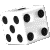Probability Theory Guide and Applications Infarom Publishing                                                                                                                                      ROPowered bySociety for Industrial and Applied Mathematics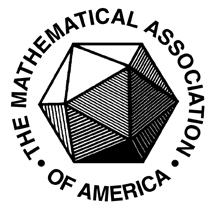Mathematical Association of AmericaUniversity of Bucharest

A project of

PhilScienceEvery one of us uses the words probable, probability or odds few times a day in common speech when referring to the possibility of a certain event happening. Whether we have math skills or not, we frequently estimate and compare probabilities, sometimes without realizing it, especially when making decisions. But probabilities are not just simple numbers attached objectively or subjectively to events, as they perhaps look, and their calculus and usage is highly predisposed to qualitative or quantitative errors in the absence of proper knowledge.
This site is a brief introduction to Probability Theory Basics and Probability Calculus, with goals of helping non-mathematicians to apply and perform probability calculus without a teacher and to stimulate them to go deeper into the notions involved.

Beyond the word probability, which in common speech usually represents a certain subjective degree of belief in the occurence of an event, there is a whole conceptual ensemble developed by Probability Theory. Moreover, the concept of probability has major philosophical and psychological implications and several scientific interpretations as well. But any of its interpretations cannot leave aside the mathematical notion.
"Call the probability of an event the ratio between the number of cases that are favorable for that event to occur and the number of all equally possible cases." - this is the simplistic definition everyone knows and stands for the definition of probability on a finite field of events.
Probability Theory extends this definition to a more complex set of events (sigma-field of events), granted with a certain mathematical structure, by building a function on this set with specific properties. This function - called probability - is in fact a measure on a field of events with values in the interval [0, 1]. Learn more about these notions in the mathematical sections of this site.Blaise Pascal
(1623 - 1662)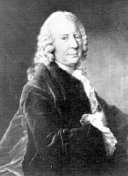James Bernoulli (1654-1705)Thomas Bayes (1702 - 1761)Pierre Laplace (1749 - 1827)Antoine Cournot (1801 - 1877)Pafnuty Chebyshev (1821 - 1894)

Probability Theory

Initially, probability theory was inspired by games of chance, especially in 17th century France, and was inaugurated by the Fermat–Pascal correspondence.    However, its complete axiomatization had to wait until Kolmogorov’s Foundations of the Theory of Probability in 1933. Over time, probability theory found several models in nature and became a branch of Mathematics with a growing number of applications. In Physics, probability theory became an important calculus tool at the same time as Thermodynamics and, later, Quantum Physics.
It has been ascertained that determinist phenomena have a very small part in surrounding nature. The vast majority of phenomena from nature and society are stochastic (random).Their study cannot be deterministic, and that is why hazard science was raised as a necessity.
Probability theory deals with the laws of evolution of random phenomena. Here are some examples of random phenomena:
1.  The simplest example is the experiment involving rolling a die; the result of this experiment is the number that appears on the upper side of the die. Even though we repeat the experiment several times, we cannot predict which value each roll will take because it depends on many random elements like the initial impulse of the die, the die’s position at the start, characteristics of the surface on which the die is rolled, and so on.
2.  A person walks from home to his or her workplace each day. The time it takes to walk that distance is not constant, but varies because of random elements (traffic, meteorological conditions, and the like).
3.  We cannot predict the percentage of misfires when firing a weapon a certain number of times at a target.
4.  We cannot know in advance what numbers will be drawn in a lottery.
In these experiments, the essential conditions of each experiment are unchanged.
All variations are caused by secondary elements that influence the result of the experiment. Among the many elements that occur in the studied phenomena, the theory focuses only on those that are decisive and ignore the influence of secondary elements. This method is typical in the study of physical and mechanical phenomena as well as in technical applications.The randomness and complexity of causes require special methods of study of random phenomena, and these methods are elaborated by probability theory.
The application of mathematics in the study of random phenomena is based on the fact that, by repeating an experiment many times in identical conditions, the relative frequency of a certain result (the ratio between number of experiments having one particular result and total number of experiments) is about the same, and oscillates around a constant number. If this happens, we can associate a number with each event; that is, the probability of that event. This link between structure (the structure of a field of events) and number is the equivalent of the mathematics of the transfer of quality into quantity. The problem of converting a field of events into a number is equivalent to defining a numeric function on this structure, which has to be a measure of the possibility of an event occurring. Because the occurrence of an event is probable, this function is named probability.

Probability theory can only be applied to phenomena that have a certain stability of the relative frequencies around probability (homogeneous mass phenomena). This is the basis of the relationship between probability theory and the real world and daily practice. So, the scientific definition of probability must first reflect the real evolution of a phenomenon. Probability is not the expression of the subjective level of man’s trust in the occurrence of the event, but the objective characterization of the relationship between conditions and events, or between cause and effect. The probability of an event makes sense as long as the set of conditions is left unchanged; any change in these conditions changes the probability and, consequently, the statistical laws governing the phenomenon.
There are almost no scientific fields in which probability theory is not applied. Also, sociology uses the calculus of probabilities as a principal tool. Moreover, some commercial domains are based on probabilities (insurance, bets, and casinos, among others).

Probability calculus
To perform probability calculus means to find the numerical probability of an event, by applying properties of probability and working the calculations for the specific parameters of the respective application or problem.
You don't have to be an expert or mathematician for being able to do probability calculus for finite applications and you don't have to go deeper in the notions of probability theory. The probability calculus skills can be developed through algorithmic procedures. The only things to know priorly are the main definitions and a set of formulas. Some combinatorial calculus skills are welcome. Besides this minimal knowledge of probability theory and combinatorics, the only requirement for the non-mathematician solver is to have a good command of the four arithmetic operations between real numbers and of basic algebraic calculus.
Theoretically, any probability calculus problem, no matter how complex, can be unfolded in successive elementary applications that use basic formulas, but sometimes finishing the calculus can be very laborious or even impossible, not to mention the high risk of the occurrence of errors during a long succession of calculations. The use of combinatorics and even of classical probability repartitions can often solve such problems simply and elegantly, whereas the step-by-step approach is much too laborious and is predisposed to calculation errors.
Every solution of a probability application submits to a basic algorithm, which basically ensures the correctness of framing and approach to the calculus problem and of the application of the theoretical results as well. Even though the methods of solving a problem can be multiple, all procedures are applied on the basis of this general algorithm, which is valid for any finite or discrete probability application. The solution algorithm consists of three main stages: framing the problem (establishing the probability field attached to an experiment, textually defining the events to be measured); establishing the theoretical procedure (choosing the solving method, selecting the formulas to use); and the calculus (numerical or combinatorial calculus and the applications of formulas).
As mentioned above, probability calculus was developed to answer questions on random phenomena, including in gambling. In what's possibly the most popular recreational activity today, gambling (especially games like poker, roulette, blackjack, slots, or lottery), probability theory is ubiquitous. Gambling strategy is erected on probability theory, and players wallow in discussions on this classical subject.

 Sources Check our Books section for good books explaining the probability concept and its interpretations and applications for non-mathematicians. Their goals are to help the reader understand what probability really means, to teach the reader how to rigorously perform and apply the probability calculus, even without a solid mathematical background, and to stimulate the reader to go deeper into the notions involved. You will find there solid A to Z teaching material about Probability Theory and the practical person can find all the tools needed to apply and perform probability calculus without a teacher. You may also browse the sections of this website for the probability theory basics with applications, including in gambling. We also provide online gambling-math courses and a gambling-math counseling service.Andrei Markov
(1856 - 1922)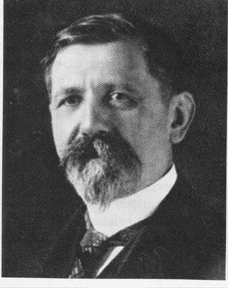Emile Borel
(1871 - 1956)Richard von Mises(1883 - 1953)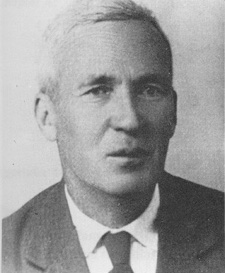Andrei N. Kolmogorov
(1903 - 1987)